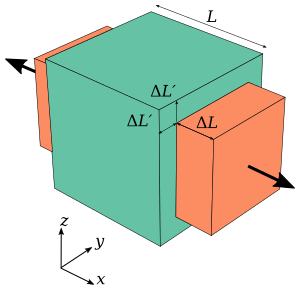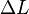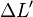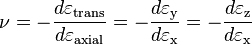# Poisson's ratio facts for kids

Kids Encyclopedia Facts

Poisson's ratio (letter v) is a measure of the contraction that happens when an object is stretched. This contraction is perpendicular to the stretching force. It can also expand as the object is compressed in a perpendicular direction.Figure 1: A cube with sides of length L of an isotropic linearly elastic material subject to tension along the x axis, with a Poisson's ratio of 0.5. The green cube is unstrained, the red is expanded in the x direction by$\Delta L$ due to tension, and contracted in the y and z directions by$\Delta L'$.

For example, if a block is being stretched as shown in the image to the right, the equation for the poisson's ratio will be:$\nu = -\frac{d\varepsilon_\mathrm{trans}}{d\varepsilon_\mathrm{axial}} = -\frac{d\varepsilon_\mathrm{y}}{d\varepsilon_\mathrm{x}}= -\frac{d\varepsilon_\mathrm{z}}{d\varepsilon_\mathrm{x}}$

Poisson's ratio ranges from 0.0-0.5 for common materials, though for materials with certain structures, can be as low as -1. A material with a Poisson's ratio close to 0 (like cork) can be stretched a lot in the axial direction without changing much at all in the transverse, where as pulling on a material with a high Poisson's ratio (like rubber) will cause it to become much more narrow. A material with a negative Poisson's ratio will expand in all directions as it is stretched.

## Images for kidsPoisson's ratio Facts for Kids. Kiddle Encyclopedia.